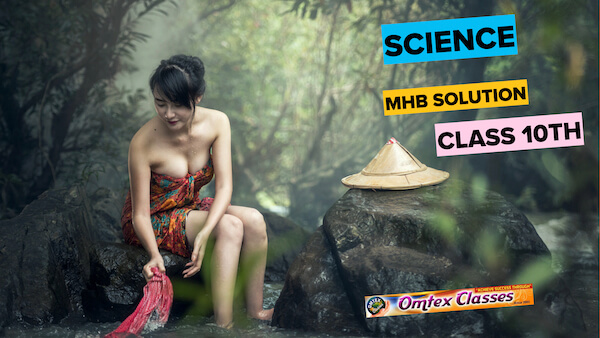### Solve the following examples. 5 cm high object is placed at a distance of 25 cm from a converging lens of focal length of 10 cm. Determine the position, size and type of the image.

Question 10.

Solve the following examples.

5 cm high object is placed at a distance of 25 cm from a converging lens of focal length of 10 cm. Determine the position, size and type of the image.

Answer:

As per the given information

Height of the object is 5 cms

Focal length f is 10 cms

Object distance u = 25 cms

Image distance v= ?

Height of the image h = ?

⇒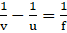⇒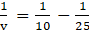⇒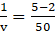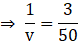V = 50/3 =16.66 cms

The image formed will be real and inverted on the other side of the lens at a distance of 16.66 cms from its optical centre.

Now

m= v/u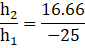Where h2 is the height of the object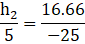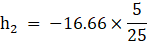⇒ h2 = 3.33cms

Therefore from above we can conclude an inverted image of 3.33cm will be formed.